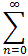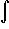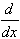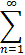#Interactive Real Analysis

Next | Previous | Glossary | Map

## 8.3. Series and Power Series

### Theorem 8.3.10: Differentiating and Integrating Power Series

Letan (x - c)n be a power series centered at c with radius of convergence r > 0. Then:
• The power series represents a continuous function for |x-c| < r
• The power series is integrable and can be integrated term-by-term for all |x - c| < r, i.e.an (x - c)n dx =an (x - c)n dx =1/n+1 an (x - c)n+1 + const
• The power series is differentiable and can be differentiated term-by-term for all |x - c| < r, i.e.an (x - c)n =an (x - c)n =n an (x - c)n-1Next | Previous | Glossary | Map